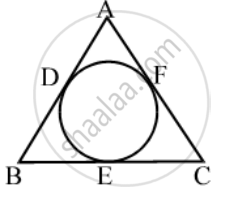Share

# In Fig., if AB = AC, prove that BE = EC - CBSE Class 10 - Mathematics

ConceptCircles Examples and Solutions

#### Question

In Fig., if AB = AC, prove that BE = EC#### Solution

Since tangents from an exterior point to a circle are equal in length.

∴ AD = AF [Tangents from A]

BD = BE [Tangents from B]

CE = CF [Tangents from C]

Now,

AB = AC

⇒ AB – AD = AC – AF [Using (i)]

⇒ BD = CF ⇒ BE = CF [Using (ii)]

⇒ BE = CE [Using (iii)]

Is there an error in this question or solution?

#### Video TutorialsVIEW ALL 

Solution In Fig., if AB = AC, prove that BE = EC Concept: Circles Examples and Solutions.
S